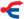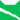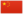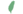本文介绍的是雷族母猫。关于芫荽的女儿“小黛西”，请参见「黛西 (独行猫)」。

Daisy雷族 (Q627)[临时][避难]黛西 Daisy  黛西

Q2379：雷族母猫，原独行猫，自《星光指路》登场的角色

## 细节

### 外貌

• 她是一只淡蓝色眼睛、:封面奶油色的长毛母猫。:猫物表她的皮毛柔软、:146脊背宽阔、:141尾巴蓬松。:210
• 她最初被描述为淡奶油棕。:227

## 登场书目

• 星光指路 (Q142)[配角][首次登场🐱]
• 黄昏战争 (Q143)[配角]
• 日落和平 (Q144)[配角]
• 预视力量 (Q152)[配角]
• 暗河汹涌 (Q153)[配角]
• 驱逐之战 (Q154)[配角]
• 天蚀遮月 (Q155)[配角]
• 暗夜长影 (Q156)[配角]
• 拂晓之光 (Q157)[配角]
• 第四学徒 (Q158)[配角]
• 战声渐近 (Q159)[配角]
• 暗夜密语 (Q160)[配角]
• 月光印记 (Q161)[配角]
• 武士归来 (Q162)[配角]
• 群星之战 (Q163)[配角]
• 学徒探索 (Q170)[配角]
• 雷影交加 (Q171)[配角]
• 天空破碎 (Q172)[配角]
• 极夜无光 (Q173)[配角]
• 烈焰焚河 (Q174)[配角]
• 风暴来袭 (Q175)[配角]
• 迷失群星 (Q3563)[配角]
• 静默冰融 (Q3579)[配角]
• 叠影重障 (Q3624)[配角]
• 暗由心生 (Q4183)[对话提及]
• 无星之地 (Q4385)[猫物闲角][对话提及]
• 迷雾明光 (Q4621)[猫物闲角]
• 河翻浪涌 (Q4643)[配角]
• 天穹将倾 (Q4799)[配角]
• 黑莓星的风暴 (Q182)[配角]
• 虎心的阴影 (Q185)[猫物闲角]
• 鸦羽的拷问 (Q186)[配角]
• 松鼠飞的希冀 (Q3561)[配角]
• 灰条的誓言 (Q4057)[猫物闲角]
• 一星的告解 (Q4826)[间接提及]
• 冬青叶的故事 (Q187)[猫物闲角]
• 雾星的征兆 (Q188)[猫物闲角]
• 叶池的祈愿 (Q191)[配角]
• 鸽翅的沉默 (Q192)[配角]
• 褐皮的族群 (Q3566)[猫物闲角]
• 黛西的至亲 (Q3629)[主人公][主要角色][视角人物]
• 斑毛的反抗 (Q4337)[猫物闲角]
• 族群的秘密 (Q199)[追溯修订]
• 族群的猫 (Q200)[对话提及]
• 族群的战争 (Q202)[配角]
• 终极指南 (Q204)[对话提及]

## 参考文献

这篇文章基于CC BY-SA 3.0许可使用了猫武士维基（英语）Daisy (TC)一文中的部分内容。